Permeability

# Permeability - Notes | Study Civil Engineering SSC JE (Technical) - Civil Engineering (CE)

 1 Crore+ students have signed up on EduRev. Have you?

Chapter 3:
Permeability

PERMEABILITY OF SOIL:
The ability of soil to allow flow of water through it is called as permeability of soil. It is very important factor for the structures which are in contact with water. Flow of water in soil takes place through void spaces, which are interconnected. Water does not flow in a straight line, but in a winding path. However in soil mechanics flow is considered to be in a straight line at an effective velocity. The velocity of flow depends on size of pores.

DARCY’S LAW
Darcy established that the flow occurring per unit time is directly proportional to the head causing flow and the area of cross-section of the soil sample but is inversely proportional to the length of the sample.
(i) Rate of flow (q)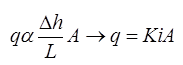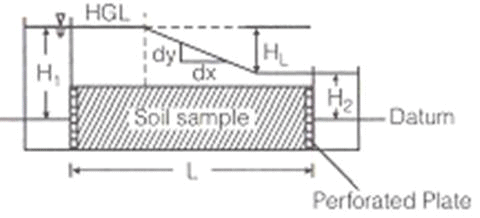Where,
q = rate of flow in m3/sec.
K = Coefficient of permeability in m/s
A = Area of cross-section of sample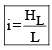Where,
HL = Head loss = (H1 - H2)
i = tanθdy/dx

(ii) Seepage velocity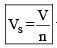Where, Vs = Seepage velocity (m3/sec) and n = Porostiy,V=discharge velocity(m/s)

(iii) Coefficiency of percolation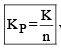where, Kp = coefficiency of percolation and n = Porosity.

-The constant head permeability test is a laboratory experiment conducted to determine the permeability of soil. The soils that are suitable for this tests are sand and gravels. Soils with silt content cannot be tested with this method.The test can be employed to test granular soils either reconstituted or disturbed.
-The objective of constant head permeability test is to determine the coefficient of permeability of a soil.

-The coefficient of permeability, k is defined as the rate of flow of water under laminar flow conditions through a porous medium area of unit cross section under unit hydraulic gradient.

The coefficient of permeability (k) is obtained from the relation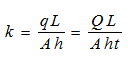Where q= discharge, Q=total volume of water, t=time period, h=head causing flow, L= length of specimen, A= cross-sectional area.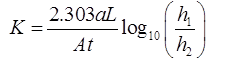where,
a = Area of tube in m2
A = Area of sample in m2
t = time in ‘sec’
L = length in ‘m’
h1 = level of upstream edge at t = 0
h2 = level of upstream edge after time ‘t’.
Falling head permeability test is useful for fine grained soil. and it is laboratory method.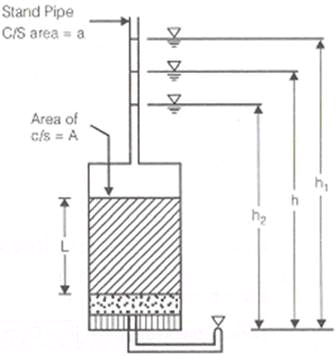KONZEY-KARMAN EQUATION: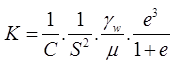where, C = Shape coefficient, ∼ 5mm for spheri cal particle
S = Specific surface area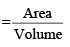For spherical particle,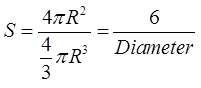R = Radius of spherical particle.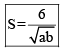When particles are not spherical and of variable size.If these particles passes through sieve of size ‘a’ and retain on sieve of size ‘b’.
e = void ratio
μ = dynamic viscosity, in (N-s/m2)
γw = unit weight of water in kN/m3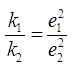ALLEN HAZEN EQUATION: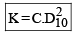Where, D10 = Effective size in cm,K is in cm/s ,C   = 100 to 150
NOTE: it is valid for particle size of soil 1 mm to 3 mm. It is valid for sand.

LIOUDENS EQUATION:
log10 KS2 = a +b.n
where, S = specific surface area
a and b are constants
N = Porosity.
Consolidation equation,K = CvMvγw
where,
CV = Coefficient of consolidation in cm2/ sec
Mv = Coefficient of volume Compressibility in cm2/N
PERMEABILITY OF A STRATIFIED SOIL
(i) Average Permeability of the soil in which flow is parallel to bedding plane.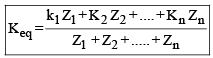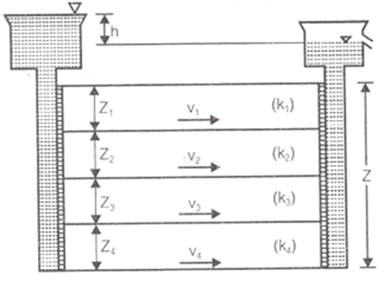(ii) Average permeability of soil in which flow is perpendicular to bedding plane.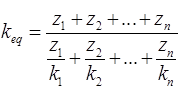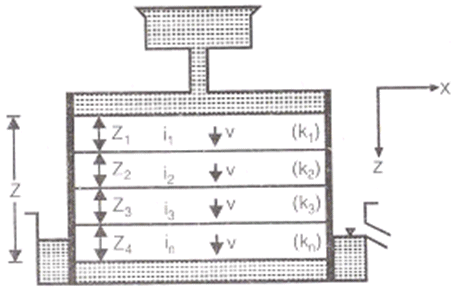(iii) For 2-D flow in X and Z direction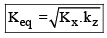iv) For 3-D flow in x, y and z direction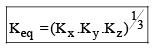COEFFICIENT OF ABSOLUTE PERMEABILITY(Ko):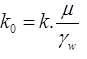The document Permeability - Notes | Study Civil Engineering SSC JE (Technical) - Civil Engineering (CE) is a part of the Civil Engineering (CE) Course Civil Engineering SSC JE (Technical).
All you need of Civil Engineering (CE) at this link: Civil Engineering (CE)

## Civil Engineering SSC JE (Technical)

2 videos|122 docs|50 tests
 Use Code STAYHOME200 and get INR 200 additional OFF

## Civil Engineering SSC JE (Technical)

2 videos|122 docs|50 tests

Track your progress, build streaks, highlight & save important lessons and more!

,

,

,

,

,

,

,

,

,

,

,

,

,

,

,

,

,

,

,

,

,

;